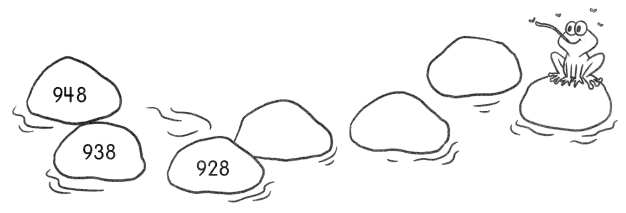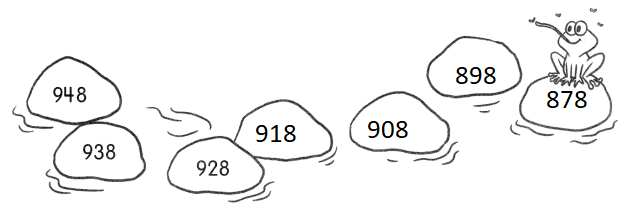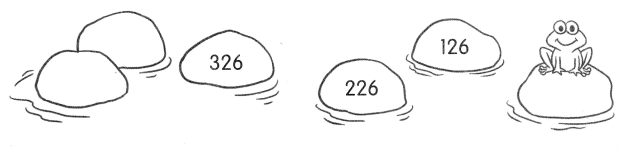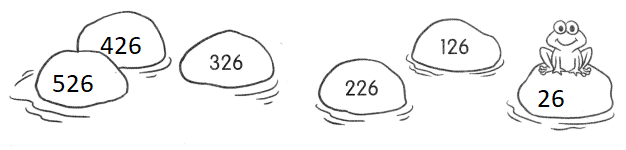# Math in Focus Grade 2 Chapter 1 Practice 1 Answer Key Counting

Go through the Math in Focus Grade 2 Workbook Answer Key Chapter 1 Practice 1 Counting to finish your assignments.

## Math in Focus Grade 2 Chapter 1 Practice 1 Answer Key Counting

Write the numbers shown by the base-ten blocks.

Example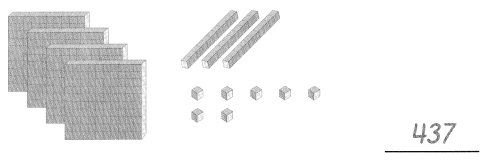Question 1.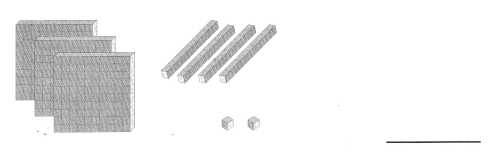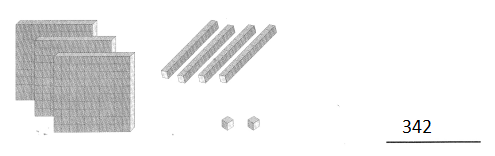Explanation:
1 cube = 100
1 line = 10
1 dot = 1
3 x 100 = 300
4 x 10 = 40
2x 1 = 2
300 + 40 + 2 = 342

Question 2.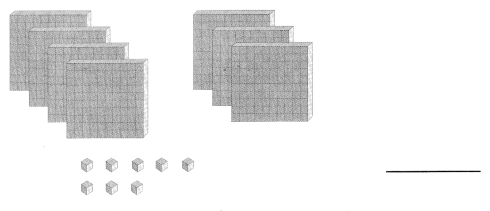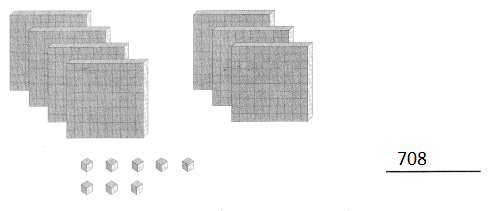Explanation:
1 cube = 100
1 line = 10
1 dot = 1
7 x 100 = 700
700 + 8 = 708

Write the numbers in words.

Example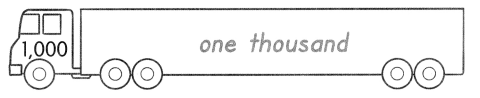Question 3.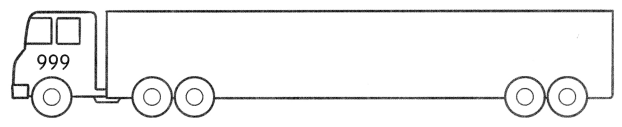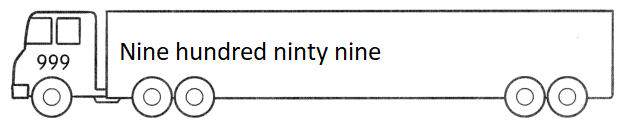Explanation:
Standard  form is converted to word form

Question 4.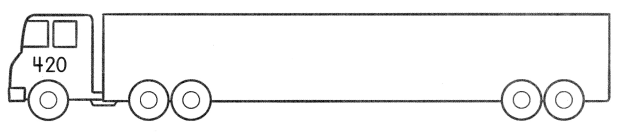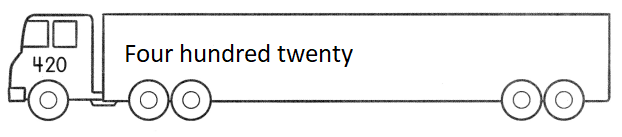Explanation:
Standard  form is converted to word form

Question 5.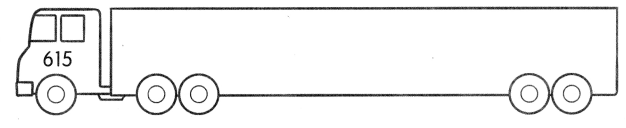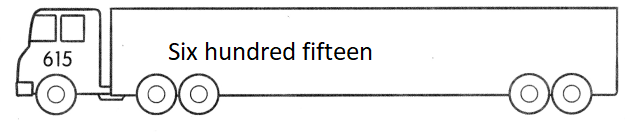Explanation:
Standard  form is converted to word form

Question 6.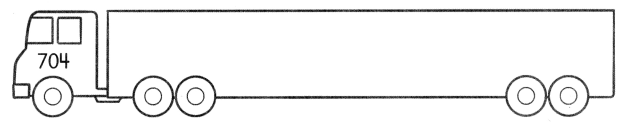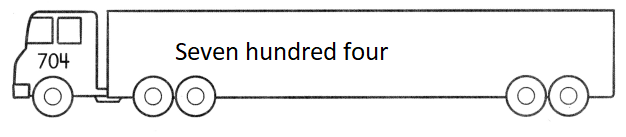Explanation:
Standard  form is converted to word form

Question 7.
Fill in the chart with the correct numbers. The first one is done for you.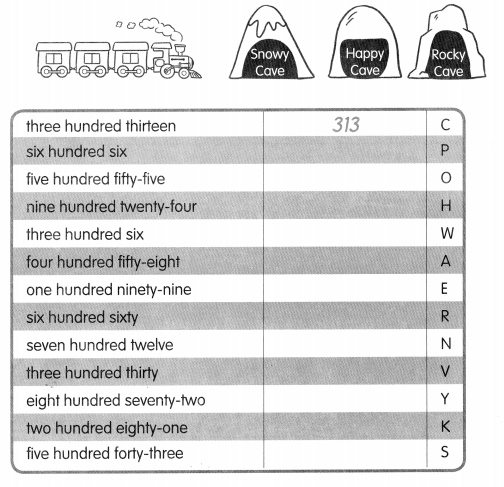Explanation: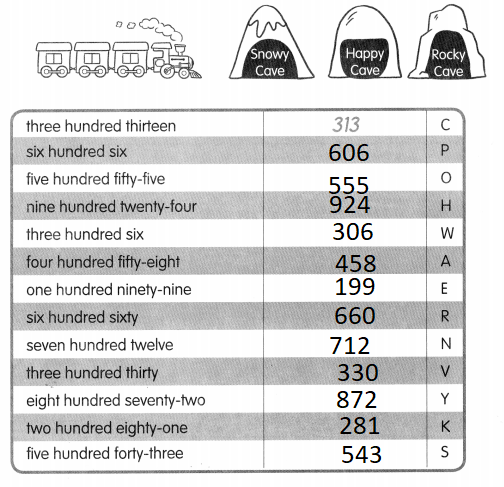Word form is converted standard form

Write the letters that match the numbers. Find which cave the train is going to. An example is shown.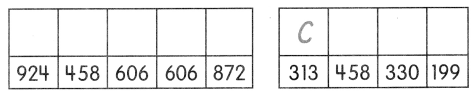Explanation: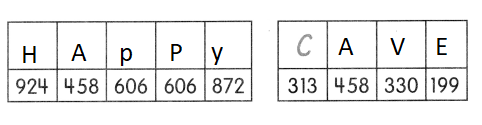With the help of answers we founded the cave

Find the missing numbers.

Question 8.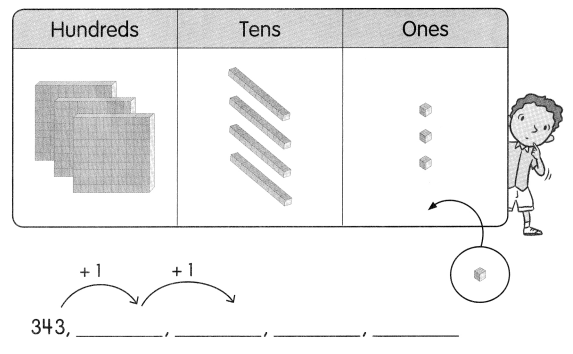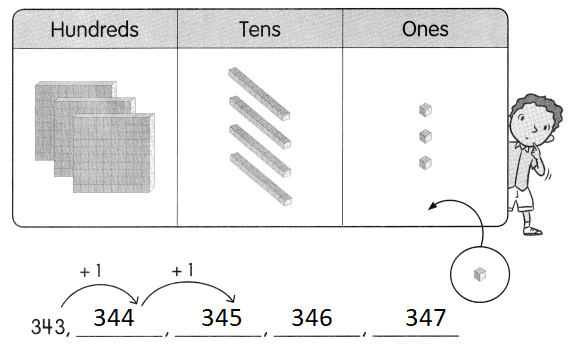Explanation:
In the above pattern 1 is added in the ones place

Question 9.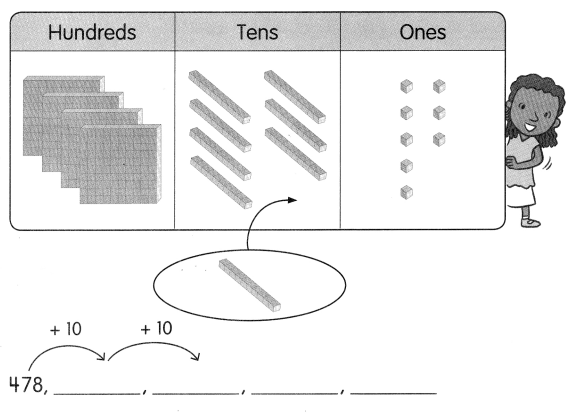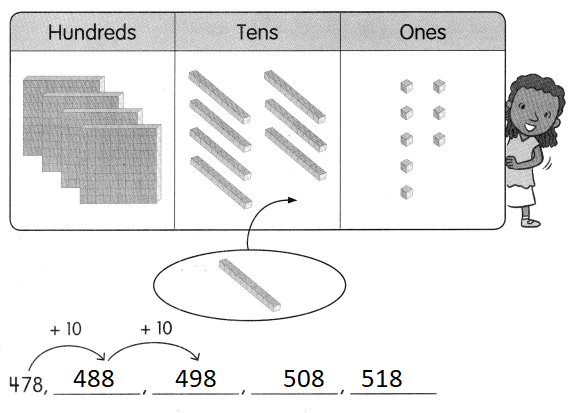Explanation:
In the above pattern 10 is added in the tens place

Find the missing numbers.

Question 10.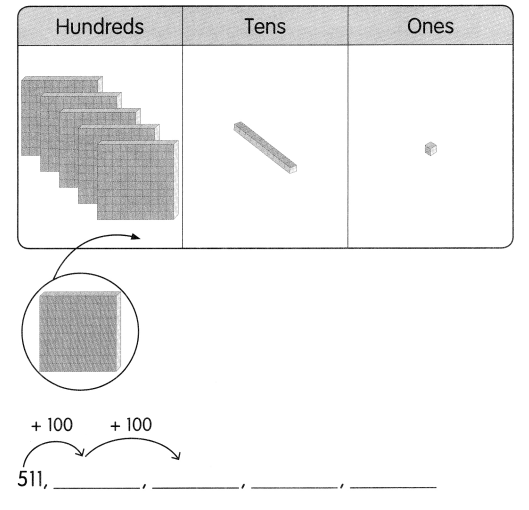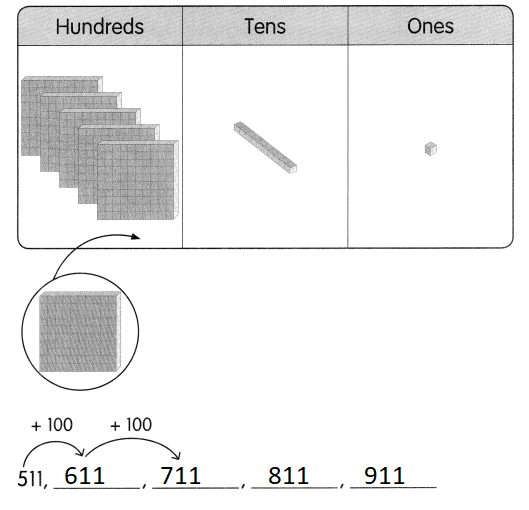Explanation:
In the above pattern 100 is added in the hundreds place

Freddy Frog loves to count.
Count with him and find the missing numbers.

Question 11.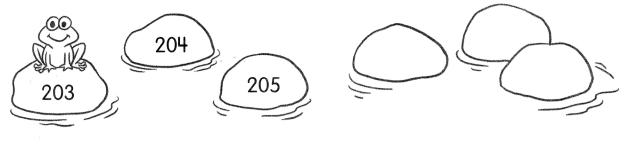Explanation:
In the above pattern 1 is added in the ones place

Freddy Frog loves t0 count.
Count with him and find the missing numbers.

Question 12.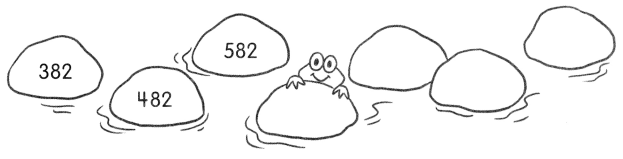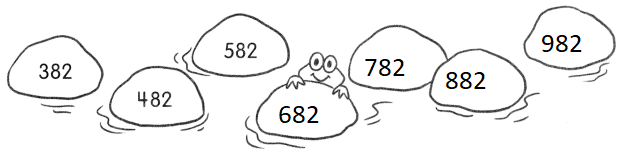Explanation:
In the above pattern 100 is added in the hundreds place

Question 13.